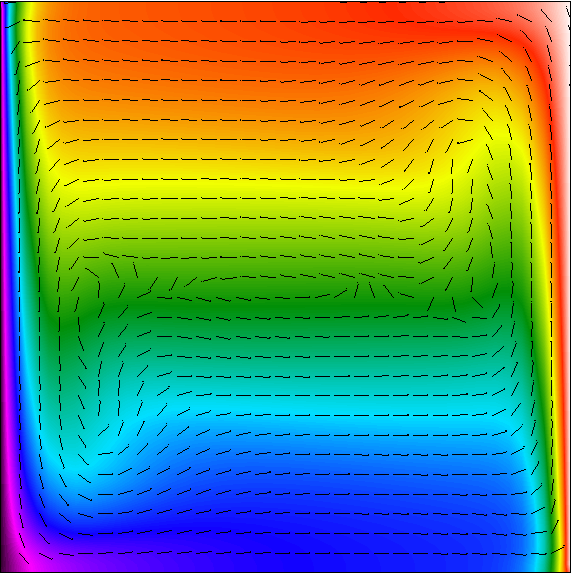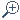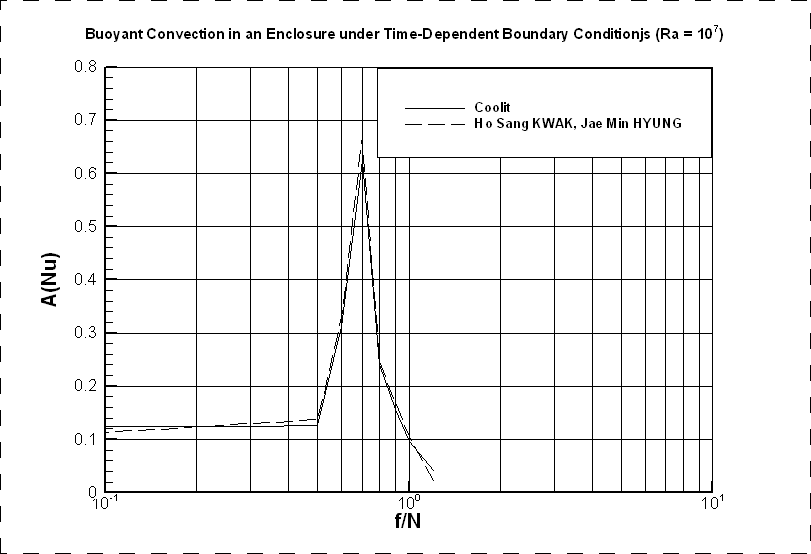` ` Gallery of real customer solutions using Coolit### Transient flow with resonance benchmark.

Courtesy of NTS Corp.

In most cases, free convection flows are unstable and hence transient. Of significant interest is the possibility of a resonance between the time-dependent forcing function (e.g. time-dependent power source) and natural frequencies of the system, which can cause significant amplification of heat transfer rates, above and beyond the ones caused by either the forcing function or the natural oscillations.

One of the fundamental models used to study the effects of resonance in free convection is a rectangular cavity. The top and bottom walls are insulated, the left wall is kept at a constant temperature, Tc, and at the right wall either the periodic heat flux, qH(t), or the temperature, TH(t), is applied. Following Kwak and Hyun (2000), we define qH(t) = q[1+e sign (sin ft)] and TH(t) = T h + DT e sin ft, where e is the amplitude of the forcing function, DT = Th  Tc , Th is the mean value T on the right (hot) wall.

The impact of periodic heating is assessed using the following function:

A(f) = [max ( f)  min ( f)] / fs,

which measures the magnitude of oscillations relative to the non-oscillating value.

We will use the Nusselt number at the vertical center plane, as the function f. Figure 1 shows a snapshot of the free convection flow in a cavity with differently heated walls. Figure 2 shows A(Nu) as a function of the forcing frequency f normalized by the Brunt-Vaisala frequency, N = (ag DT/H)0.5, where H is the linear size of the cavity. The figure results were computed using Coolit and compared to results from Kwak and Hyun (2000).

References:

HS Kwak and JM Hyun, JSME International J., Series B, V. 13, N4, pp. 532-7, 2000.Figure 1. Snapshot of the free convection flow in a cavity with differently heated walls.Figure 2. A(Nu) as a function of the forcing frequency f normalized by the Brunt-Vaisala frequency.